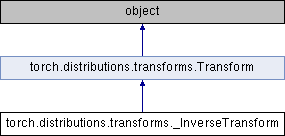Caffe2 - Python API A deep learning, cross platform ML framework
torch.distributions.transforms._InverseTransform Class Reference
Inheritance diagram for torch.distributions.transforms._InverseTransform:## Public Member Functions

def __init__ (self, transform)

def domain (self)

def codomain (self)

def bijective (self)

def sign (self)

def event_dim (self)

def inv (self)

def __eq__ (self, other)

def __call__ (self, x)

def log_abs_det_jacobian (self, x, y)Public Member Functions inherited from torch.distributions.transforms.Transform
def __init__ (self, cache_size=0)

def inv (self)

def sign (self)

def __eq__ (self, other)

def __ne__ (self, other)

def __call__ (self, x)

def log_abs_det_jacobian (self, x, y)

def __repr__ (self)Static Public Attributes inherited from torch.distributions.transforms.Transform
bijective

event_dim

## Detailed Description

```Inverts a single :class:`Transform`.